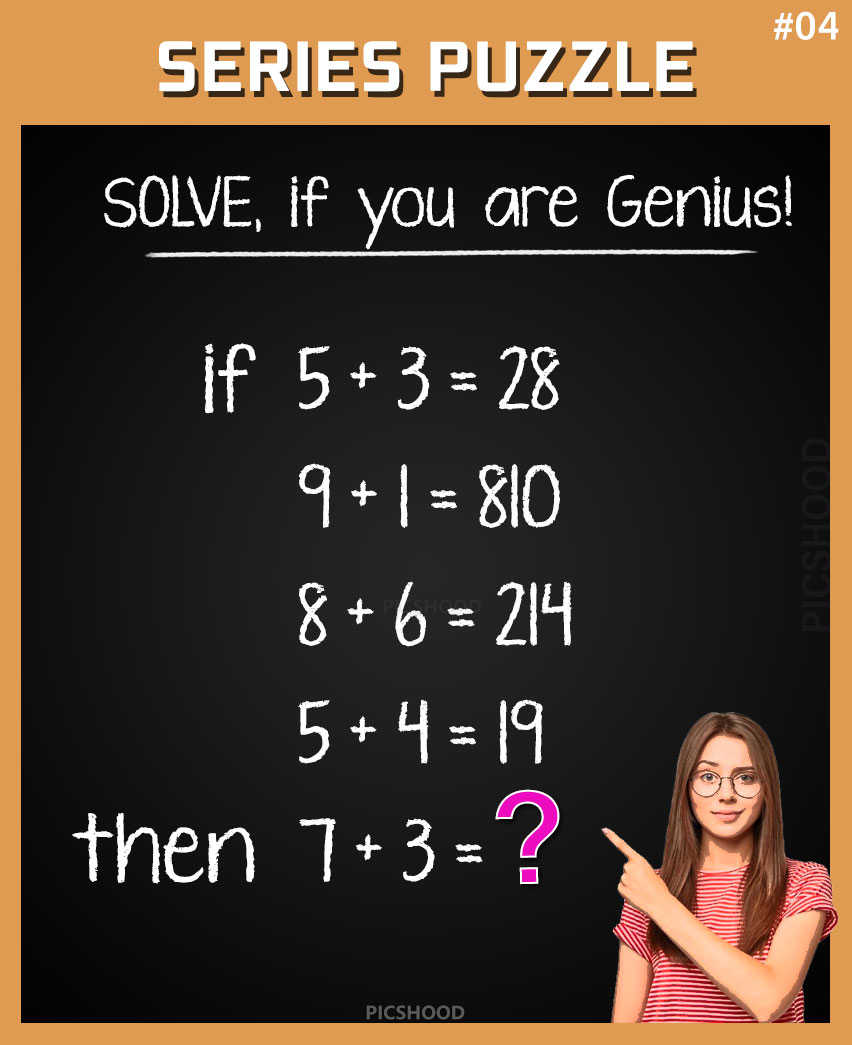This post may contain affiliate links. This means if you click on the link and purchase the item, We will receive an affiliate commission at no extra cost to you. See Our Affiliate Policy for more info.

Find if 5+3=28, then 7+3=? Only For Genius – Number Series Puzzle #4

5
5493

Genius Math Puzzles problem – Solve this if you are a Genius!

Take the challenge and solve this interesting math puzzle problem. Check the question below and share your answer via comments.

• if 5+3=28,
• 9+1=810,
• 8+6=214,
• 5+4=19,
• then 7+3=__?

Math Puzzle Image:SOLVE THIS !! GENIUS Math Puzzle Problems And Riddles With Answers & Solutions.

PATTERN:- We See Pattern, If We take First Equation, 5 + 3 = 28 ,
The Subtraction of 5 – 3 = 2, The Addition of  5 + 3 = 8,
It Completes the Digit  28,  the Subtraction gives the First Digit  2  and  Addition Gives the Second  Digit   8 .
Hence it’s Formed 28 .

And In SECOND Equation,    9 + 1 = 810,
Same Pattern as the First, the Subtraction 9 – 1 = 8,
The Addition 9 + 1 = 10,
Hence the First Digit Formed 8 And Other Last Digits Formed 10. SO, 9 + 1 = 810 .

And In THIRD Equation, 8 + 6 = 214,

The Subtraction is 8 – 6 = 2 & Addition is 8 + 6 = 14 .
So, the First Digit is 2 and Other last Digits 14. Hence Formed, 214 .

In FOURTH Equation, 5 + 4 = 19,

The Subtraction Gives 5 – 4 = 1 & Addition Gives 5 + 4 = 9 .
So, the First Digit  Formed is 1 and Second Digit Formed is 9. Hence Formed 5 + 4 = 19 .

And FINALLY, 7 + 3 = ? ,

The Subtraction of 7 – 3 = 4 & the Addition of 7 + 3 = 10 .

So, the First Digit Formed 4 and other last Digits Formed 10. Hence 7 + 3 = 410 .

Comment down below If you See Another Pattern or Formation in this Puzzles.

LIKE us on facebook and get more interesting puzzles in your timeline

Thank You !! Take Care, Enjoy!

1.Goatmaster300

810 cuz the second one is (9+1=810) 9+1=10__ 7+3=10 as well therefore 7+3=810

•Ranju

It is 411 bcz 7-3=4 and 7+3=11

•Ranju

Sorry 410 bcz 7-3=4 and 7+3=10

2.Hashmi irfan

7+3=10

3.Uzma

7+3=52